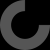# Parametric Open SCAD Timingbelts

Mar 22, 2012

### Please Login to Comment

here is a hacked together example based on something else I did a while back, you only need to add a small triangle to fill up the gap, I would extend it but my machine is in need of attention. add it in at the top of scad file to begin

//Variables

tooth_profile = "T5"; // "T2.5", "T5" , "T10"

belt_width = 4;

n = 20;

arc = 360;

//Draw

a_move = ((arc/360)*360)/n;

dia = (n2.5)/((arc/360)3.142);

function x_move(j) = dia-(sin(ja_move)dia);

function y_move(j) = dia+(cos(ja_move)dia);

function z_move(j) = 0;

function z_rot(j) = 90 + (j * a_move);

union(){

for(j = [0:n])

{

echo(j,x_move(j),y_move(j),z_move(j));

translate([x_move(j),

``````        y_move(j),

z_move(j)])``````

rotate([0,

``````    0,

a_move*j])

belt_length(profile = tooth_profile, belt_width = 10, n = 0.5);

}``````

}

I have been meaning to try this but haven't yet, but I imagine if you create an SCAD file that calls this library you could create an arc by calling a section of belt 1 tooth long over a circular or oval path. I still might have a go but you could have a look at droftarts's gear script or the 3d printable belt that I can't seem to find right now for inspiration. Derive and beat me to it.

"Stole the profiles"?! Give them back! Actually, I forgive you. Here, have them. Good idea! :-D

Well "Stole" just seemed to scan better than "used, properly attibuted, and released under same licence".

8-)